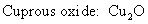##### Inorganic Chemistry For Dummies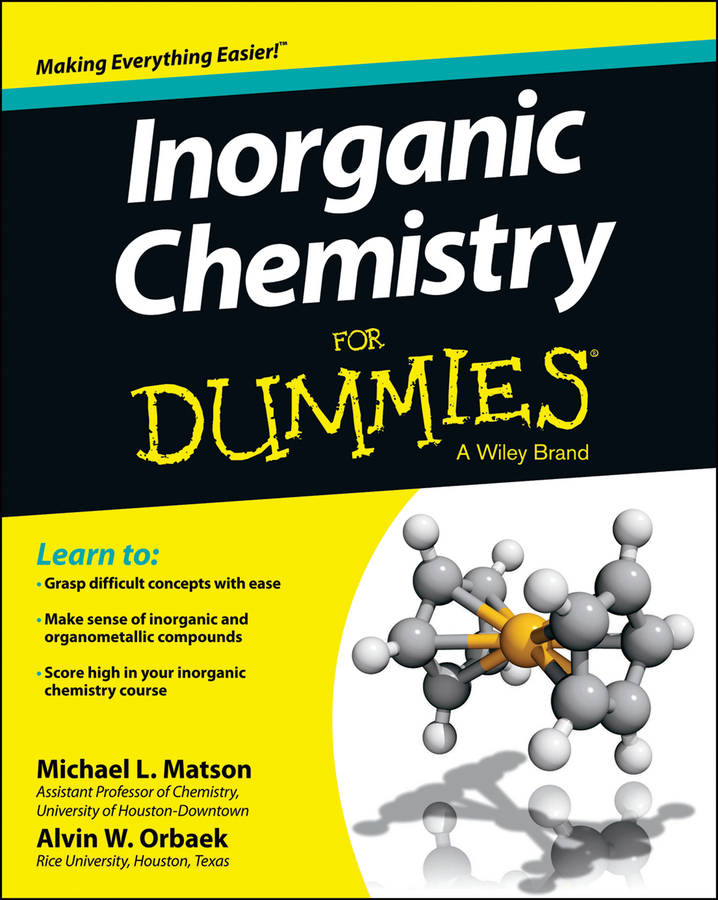When you name ionic compounds, you write the name of the metal first and then the nonmetal. Suppose that you want to name the compound that results from the reaction of lithium and sulfur. You first write the name of the metal, lithium, and then write the name of the nonmetal, adding an -ide ending so that sulfur becomes sulfide.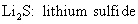Ionic compounds involving polyatomic ions follow the same basic rule: Write the name of the metal first, and then simply add the name of the nonmetal (with the polyatomic anions, it is not necessary to add the -ide ending).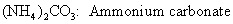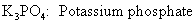When the metal involved is a transition metal with more than one oxidation state, there can be more than one way to correctly name the compound.

For example, suppose that you want to name the compound formed between the cation: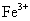and the cyanide ion: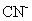The preferred method is to use the metal name followed in parentheses by the ionic charge written as a Roman numeral: Iron(III).

But an older naming method, which is still in use, is to use -ous and -ic endings. The ion with the lower oxidation state (lower numerical charge, ignoring the + or -) is given an -ous ending, and the ion with the higher oxidation state (higher numerical charge) is given an -ic ending.

So the compound can be named: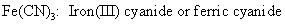Sometimes figuring out the charge on an ion can be a little challenging (and fun), so suppose you want to name the following ionic compound: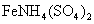The sulfate ion has a 2- charge, and from the formula you can see that there are two of them. Therefore, you have a total of four negative charges. The ammonium ion has a 1+ charge, so you can figure out the charge on the iron cation.

Because you have a 4- for the sulfates and a 1+ for the ammonium, the iron must be a 3+ to make the compound neutral. So the iron is in the Iron(III), or ferric, oxidation state. You can name the compound: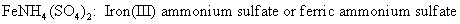And, finally, if you have the name, you can derive the formula and the charge on the ions. For example, suppose that you’re given the name cuprous oxide. You know that the cuprous ion is: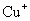The oxide ion is: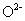Applying the crisscross rule, you get the following formula: